﻿ Solution to Collatz ConjecturePublications are Open
Access in this journal
Article Versions
Export Article
• Normal Style
• MLA Style
• APA Style
• Chicago Style
Research Article
Open Access Peer-reviewed

### Solution to Collatz Conjecture

Abhijit Manohar
American Journal of Applied Mathematics and Statistics. 2021, 9(3), 107-110. DOI: 10.12691/ajams-9-3-5
Received August 30, 2021; Revised October 04, 2021; Accepted October 12, 2021

### Abstract

Collatz Conjecture, one of the unsolved problems in mathematics is that for any positive integer, the positive integer is multiplied by 3 and 1 is added if odd, divided by 2 if even. This process is repeated, and the sequence of numbers finally reaches 1. Collatz Conjecture is notoriously escaped all attempted proofs. This paper presents a solution to Collatz Conjecture with a statistical and logical/ mathematical proof. The article demonstrates why Collatz function cannot enter an iterative infinite loop and the function will reach 1 for all positive integers.

### 1. Introduction

Henri Poincaré, the great French mathematician, who was strongly opposed to formal methods in mathematics, wrote in 1908: “It is difficult to admit that the word if acquires, when written ⊃, a virtue it did not possess when written if.” 1. This article is written logically and mathematically, in the process of arriving at the proof.

In arithmetic, the Collatz Conjecture is defined as follows,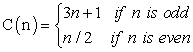The function oscillates for odd and even numbers and ultimately reaches 1. Empirically, the Conjecture is verified for numbers less than 268 2. Collatz function is depicted in Figure 3 and whenever Collatz function is discussed it specifically means the functions like shown in Figure 3.

Because of the combination of increasing odd function and decreasing even function the Collatz function oscillates until it reaches 1. Another function for example like,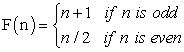will continuously decrease to 1 while a function like,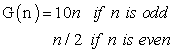will continuously increase unbounded as the increasing function is increasing faster than decreasing function and none of the numbers on the 10n function fall on exponents of 2 which only have 2, 4, 6, 8 in the unit’s place while 10n will generate a number with 0 in the unit’s place. The only exception that can occur is if the pair of function get into an infinitely recursive loop.

### 2. Proposed Solution

Even 1 are even numbers within the range 1 – 100 that can only be divided once before the quotient is an odd number. Even 2 are numbers withing the same range, that can be divided twice before the quotient is an odd number. The range 1 – 100 has up to Even 6 numbers. The Table 2 shows the frequency of occurrence of each of these numbers in the range 1 – 100. Odd 1 cannot be followed by Odd 2 as 3n + 1 will always give even number.

The weighted sum of the following frequencies is given by,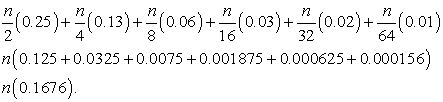These 50 even numbers are working against the 50 odd numbers which are necessarily Odd 1 only. Odd 2 and above are not possible. This is the central idea of this article. The Collatz function statistically works with Odd 1 increasing function (3n + 1) with the Weighted Even (0.1676n) Decreasing Function. The net effect is that the function statistically moves in the decreasing direction. Because the even function is statistically strong, the Collatz function eventually moves down finding smaller numbers. As the smaller even numbers are a subset of smaller numbers the Collatz function finds smaller even numbers. Hence the Collatz function decreases to the smallest even number 2 followed by 1.

The product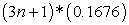is called the Collatz Product for the given range. The Collatz Product is less than value of n (for all n >2) for range 1 – 100. Which means, statistically, the Collatz function as a twice tendency to decrease the function than to increase it. The vector of function points in the negative direction. Probabilistically, the Collatz function reaches smallest even number (followed by 1).

For the range 1 – 200 the Collatz product becomes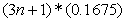which is less than the Collatz Product for the range 1 – 100 because Weighted Even Decreasing Function continuously decreases for higher range. In general, the Collatz Product for a range should remain lower than n for the dual function to collapse to 1. Logically, Collatz Product will continuously decrease as higher even functions show up for the loss of lower even functions and the value of Weighted Even Decreasing Function becomes smaller. However, larger numbers, of course will take longer runs to reach 1.

The Collatz function does not repeat numbers in the Collatz sequence as proven in section 4.

### 3. Alternating Collatz Odd and Even Function

Two examples of Collatz function which produce Odd 1 and Even 1 number sequence only are shown in Figure 3. The purpose of analysing this particular function was to see if there exist a number for which the Collatz function continuously produces an increasing curve. Of course, there do exist other combinations such as Odd 1 and Even 1, 2, 3 etc functions which will produce a sequence longer and with a higher maximum value than alternating odd and even function. But the analysis was aimed at finding the distribution of these alternating odd and even functions to check if Collatz Conjecture can be violated

• Figure 2. Example run of alternating function eventually reaching 1

It was found that the alternate length of sequence before departure from alternate Odd 1 and Even 1 is related to the trial number as shown in table above. The trial number increase as 2n + 2 for every 2 units increase in alternate length. However, after departure from alternate Odd 1 and Even 1, the function, probabilistically, eventually reaches 1 as the Collatz Product is less than n for all n > 2. Is it possible to have this alternate Odd 1 and Even 1 sequence length to reach infinity? The trial number, in that case would be larger than the sequence length.

• Figure 3. Example Collatz Functions starting from any positive integer n

### 4. Iterative Loop

There appear to be no cases (as shown in the Figure 4 - left) where Collatz Conjecture can be proven to be false for function xn + y = n if 0 ≤x≤1 and x + y ≥ 1 and 1 – x < y and 3 & 5 are factors of x and 5 but not 3 is a factor of y and 1 – x is a factor of 5 but not 3 and because it is impossible xn + y = n to be true with above conditions, the Collatz function cannot enter an iterative loop. The (1 - x) and y when considered without decimal point, we get integers which are not divisible by 2,3 but divisible by 5 and always ending in 5. However, each (1 - x) and y have at least 1 prime factor other than 2,3 and 5 which is not in the other. Consider a real Collatz function 0.6328125n + 0.5078125. 3671875 has prime factor 47 (other than 5) while 5078125 has prime factor 13, 23 and 139 (other than 5). So, 47 and 13 are different prime factors. The other number (y) always has at least 1 prime factor different than the number (1 - x). Or, if the number of prime factors of y and (1 - x) is same, it is possible that there is a pair of prime factors (one of them can be 5) of y and (1 - x) that of course doesn’t divide into an integer. Hence, y and (1 - x) cannot be divided to obtain an integer. In other words, y/ (1 - x) will be a fraction and not an integer. Any number whether, integer or fraction except 1, if we add 1 to it and perform the division of the 2 numbers, the quotient will of course not be an integer because for the quotient to be 2 (other than 1 for which the 2 numbers need to be the same) at the most the numbers need to be double of one another. Which is not possible except 1 and 2. This is also true when both the numbers are multiplied by 3 before adding 1 to one of them. So, y/x even though different numbers can never be an integer regardless of how many times you divide them with 2. Hence, y and (1 - x) also do not divide into an integer and so -y/ (x - 1) cannot be an integer either as multiplying two numbers with 3 and adding 1 to one of the numbers and subtracting 1 from the other, even after dividing by 2 any number of times still does not give an integer value except for trivial solution 0.75n + 0.25 = n (n = 1), regardless of how many times the coefficient and constant number is divided by 2. So, xn + y = n is not possible in the Collatz sequence.

It only works in a hypothetical non- real trivial example 0.75n + 1.25. The coefficient term is associated with a unique constant number, so 0.75n is known to be associated with 0.25 not 1.25. So, 0.75n + 1.25 is incorrect, impossible Collatz function. So, Collatz conjecture cannot repeat numbers in the sequence.

### 5. Conclusion

The intention in writing this paper is not to summarize or build upon the already existing development regarding the solution to “Collatz Conjecture” but to attempt to find a novel solution from scratch. This article has not mentioned the other developments regarding the solution to Collatz Conjecture. This conjecture is assumed to be not completely solved as of today.

The article, in section 2 showed that the dual function ultimately decreases in all cases due to Collatz Product value being always lower than n (n > 2). The Collatz Product will continuously decrease for larger ranges. The lower Collatz Product (always lower than n > 2) indicates a probability vector pointing in negative direction causing the function to probabilistically decrease over the runs.

The function cannot, as shown in section 4, repeat numbers in the sequence as it is impossible in case of Collatz function to arrive at n again starting from n. Because the function is probabilistically decreasing and non-iterative, non-repeating, smallest even number (followed by 1 of course) will be reached. Collatz Conjecture is true and is true for the proofs and logical explanations presented in the paper.

### References

  Kossak, Roman. Mathematical Logic: On Numbers, Sets, Structures and Symmetry. Springer Graduate Texts in Philosophy, Volume 3, Springer International Publishing AG, part of springer nature 2018, ISBN 978-3-319-97298-5. In article  Honner, Patrick. (2020). The simple Math problem we still cannot solve. Quantamagazine. The Simple Math Problem We Still Can’t Solve | Quanta Magazine. In articleThis work is licensed under a Creative Commons Attribution 4.0 International License. To view a copy of this license, visit http://creativecommons.org/licenses/by/4.0/